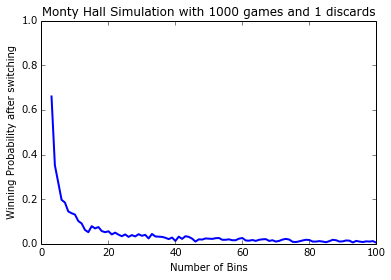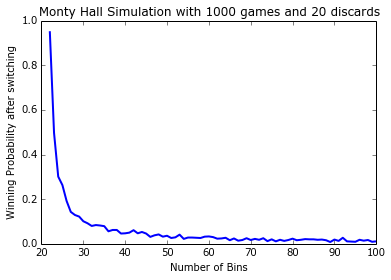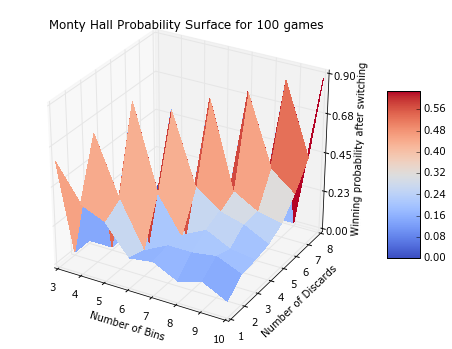# General Monty Hall Game Simulation¶

### Descriptions are available at metalearner.org¶

In :
# -*- coding: utf-8 -*-
# Author: Ehsan M. Kermani, [email protected]
# Website: metalearner.org

"""
Monty Hall Game Simulation [[http://en.wikipedia.org/wiki/Monty_Hall_problem]]
"""

import numpy as np
from numpy.random import RandomState
from random import sample
from mpl_toolkits.mplot3d import Axes3D
from matplotlib import cm
from matplotlib.ticker import LinearLocator, FormatStrFormatter
import matplotlib.pyplot as plt
%matplotlib inline

class MontyHall(object):
"""
Creates simulation game object

Parameters:
----------
n_games:    int         Number of games
n_bins:     int         Number of bins
switch:     boolean     Switch or not
seed:       int         Seed number
"""

def __init__(self, n_games, n_bins, n_discards, switch=False, seed=123):
self.n_games = n_games
self.n_bins = n_bins
if 1 <= n_discards <= n_bins-2:
else:
raise ValueError("n_discards must be between 1 and n_bins-2")
self.switch = switch
self.seed = seed

def set_prize(self):
""" Set prize randomly for each game with fixed RandomState """
prng = RandomState(self.seed)
return prng.randint(0, self.n_bins, self.n_games)

def player_first_choice(self):
""" Player first choice in each game with fixed
RandomState to get same numbers in different calls
"""
prng = RandomState(2 * self.seed)
return prng.randint(0, self.n_bins, self.n_games)

def player_final_choice(self):
""" Player final choice after discarding some options by host"""
if not self.switch:
return self.player_first_choice()
else:
opts = list(range(self.n_bins))
final = self._col_choice(opts, arr, 1)
return final

""" Host choice for removing n_discards bins"""
if self.switch:
opts = list(range(self.n_bins))
arr = np.vstack([self.set_prize(), self.player_first_choice()])
return disc

def _col_choice(self, opts, arr, n_disc):
""" Possible choices per game"""
try:
res = np.apply_along_axis(
lambda x:
sample([v for i, v in enumerate(opts)
if i not in set(x)], n_disc),
axis=0,
arr=arr)
return res
except:
print(self.n_discards, 'must be less than', self.n_bins - 1)

def score(self):
""" Calculate the number of wins"""
return np.sum(self.player_final_choice() == self.set_prize())

def proba(self):
""" Compute the winning probability"""
return self.score() / self.n_games

def __str__(self):
if not self.switch:
return 'Probability of winning if not switching: %.4f' \
% self.proba()
else:
return 'Probability of winning if switching: %.4f' \
% self.proba()

if __name__ == '__main__':

""" Compute simulation probability of n_games with n_bins
"""
switch=switch)
return g.proba()

""" Simulation 2D plot """
Y = np.array([simulation_proba(n_games, b, n_discards, switch)
for b in X])
plt.plot(X, Y, linestyle='-', color='b', lw=2)
plt.xlabel('Number of Bins')
if switch:
plt.ylabel('Winning Probability after switching')
else:
plt.ylabel('Winning Probability if not switching')
plt.title("Monty Hall Simulation with %d games and %d discards"
plt.ylim(0.0, 1.0)
# save must be before show
plt.savefig('simulation_2dplot.png', dpi=300)
plt.show()

""" Simulation 3D plot"""
X = np.array(range(3, max_bins))
X_grid, Y_grid = np.meshgrid(X, Y)
X_grid_utri, Y_grid_utri = X_grid[triu_idx], Y_grid[triu_idx]

vect_simulation_proba = np.vectorize(simulation_proba)
Z = vect_simulation_proba(n_games, X_grid_utri, Y_grid_utri, switch)
nZ[triu_idx] = Z

fig = plt.figure(figsize=(8, 6))
ax = fig.gca(projection='3d')
surf = ax.plot_surface(X_grid, Y_grid, nZ, rstride=1, cstride=1,
cmap=cm.coolwarm, linewidth=0,
antialiased=False)
ax.set_zlim = (0.0, 1.0)
ax.set_xlabel('Number of Bins')
if switch:
ax.set_zlabel('Winning probability after switching')
else:
ax.set_zlabel('Winning probability if not switching')
ax.zaxis.set_major_locator(LinearLocator(5))
ax.zaxis.set_major_formatter(FormatStrFormatter('%.02f'))
ax.set_title('Monty Hall Probability Surface for %d games' % n_games)

fig.colorbar(surf, shrink=0.5, aspect=5)

fig.savefig('3d_simulation.png', dpi=300)  # save must be before show
plt.show()

In :
simulation_proba(100000, 3, 1, switch=True)

Out:
0.66413999999999995
In :
simulation_proba(100000, 3, 1, switch=False)

Out:
0.33585999999999999
In :
simulation_proba(100000, 4, 1, switch=True)

Out:
0.37542999999999999
In :
simulation_proba(100000, 4, 2, switch=True)

Out:
0.75002999999999997
In :
simulation_2dplot(1000, 101, 1, switch=True)In :
simulation_2dplot(1000, 101, 20, switch=True)In :
simulation_3dplot(100, 11, 9, switch=True)